## Ward-Takahashi 等式

$k_{\mu}\mathcal{M}^{\mu}(k) = 0 \tag{1}$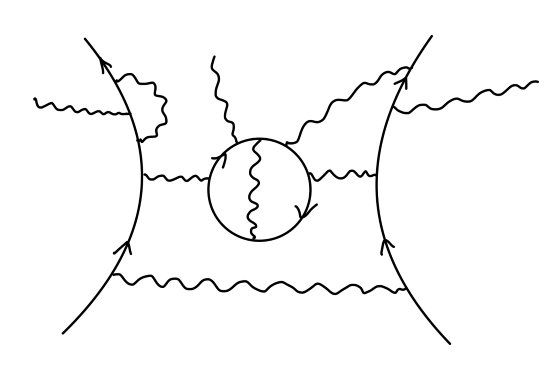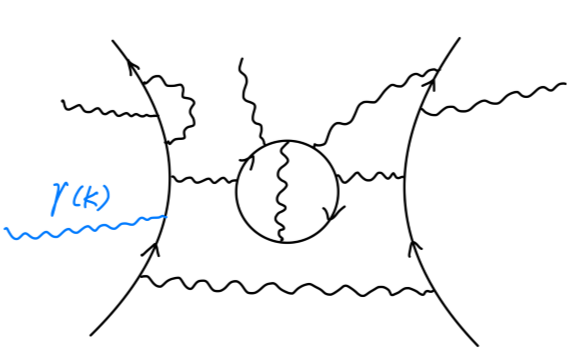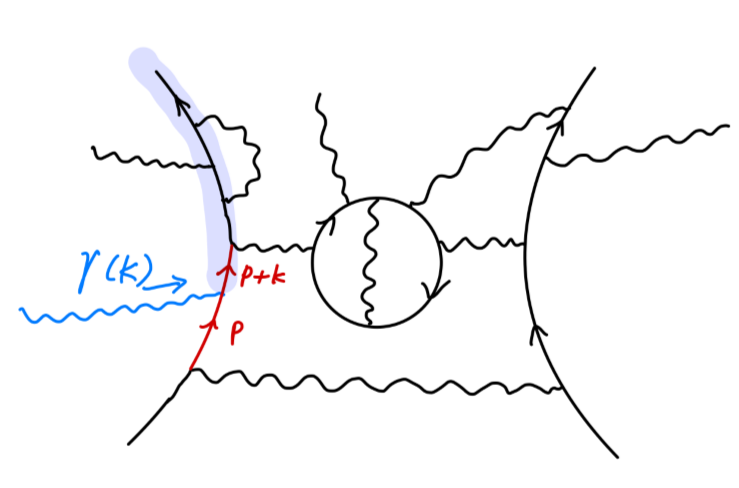\begin{aligned} \frac{i}{\cancel{p}-m}&\rightarrow k_{\mu}\cdot \frac{i}{\cancel{p}-m} (-ie\gamma^{\mu}) \frac{i}{\cancel{p} + \cancel{k}-m}\\ &= e \frac{i\cancel{k}}{(\cancel{p}-m)(\cancel{p} + \cancel{k}-m)}\\ &= e(\frac{i}{\cancel{p}-m}-\frac{i}{\cancel{p} + \cancel{k}-m}) \end{aligned}

Ward-Takahashi 的一个重要想法是：通过将所有有贡献的 $\mathcal{M}_0$ 相加，或是将外光子插在所有可能位置的费曼图相加，都可以得到 $\mathcal{M}(k)$。现在将 $\epsilon_{\mu}(k)$ 替换为 $k_{\mu}$，以上两种思路实际上为：

$\sum_{insertion\ points} (k_{\mu}\mathcal{M}^{\mu}(j)) = e\sum \mathcal{M}_0$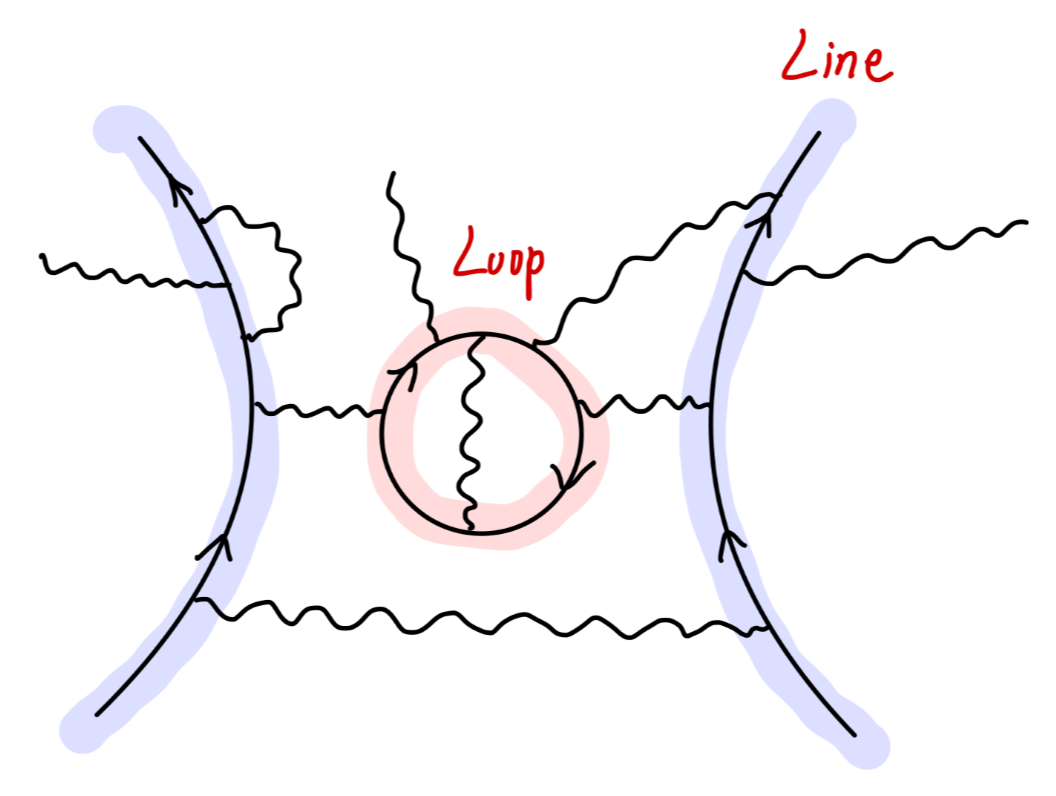• 与外部相连，贯穿整个费曼图
• 形成费米子圈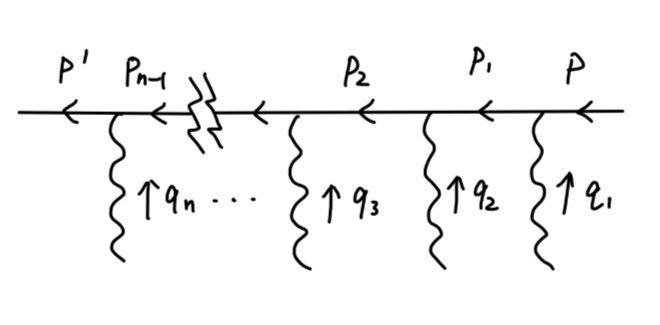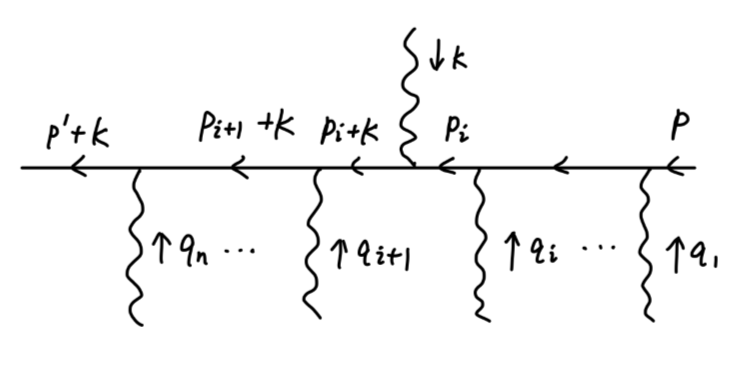$-ie\cancel{k} = -ie[(\cancel{p}_i+\cancel{k}-m)-(\cancel{p}_i-m)]$

\begin{aligned} \frac{i}{\cancel{p}_i+\cancel{k}-m}(-ie\cancel{k})\frac{i}{\cancel{p}_i-m} &= \frac{i}{\cancel{p}_i+\cancel{k}-m}(-ie[(\cancel{p}_i+\cancel{k}-m)-(\cancel{p}_i-m)])\frac{i}{\cancel{p}_i-m}\\ &= e(\frac{i}{\cancel{p}_i-m}-\frac{i}{\cancel{p}_i+\cancel{k}-m})\\ \end{aligned}

$k_{\mu}\mathcal{M}^{\mu}_i(k) = \cdots(\frac{i}{\cancel{p}_{i+1}+\cancel{k}-m})\gamma^{\lambda_{i}+1}(\frac{i}{\cancel{p}_i-m}-\frac{i}{\cancel{p}_i+\cancel{k}-m})\gamma^{\lambda_i}(\frac{i}{\cancel{p}_{i-1}-m})\gamma^{\lambda_{i}-1}\cdots$

$k_{\mu}\mathcal{M}^{\mu}_{i-1}(k) = \cdots(\frac{i}{\cancel{p}_{i+1}+\cancel{k}-m})\gamma^{\lambda_{i}+1}(\frac{i}{\cancel{p}_{i}+\cancel{k}-m})\gamma^{\lambda_i} (\frac{i}{\cancel{p}_{i-1}-m}-\frac{i}{\cancel{p}_{i-1}+\cancel{k}-m})\gamma^{\lambda_i}\cdots$

$\sum_i k_{\mu}\mathcal{M}^{\mu}_i(k) = e (\frac{i}{\cancel{p}-m})\cdots(\frac{i}{\cancel{q} - \cancel{k}-m}) - e (\frac{i}{\cancel{p} + \cancel{k}-m})\cdots(\frac{i}{\cancel{q}-m})$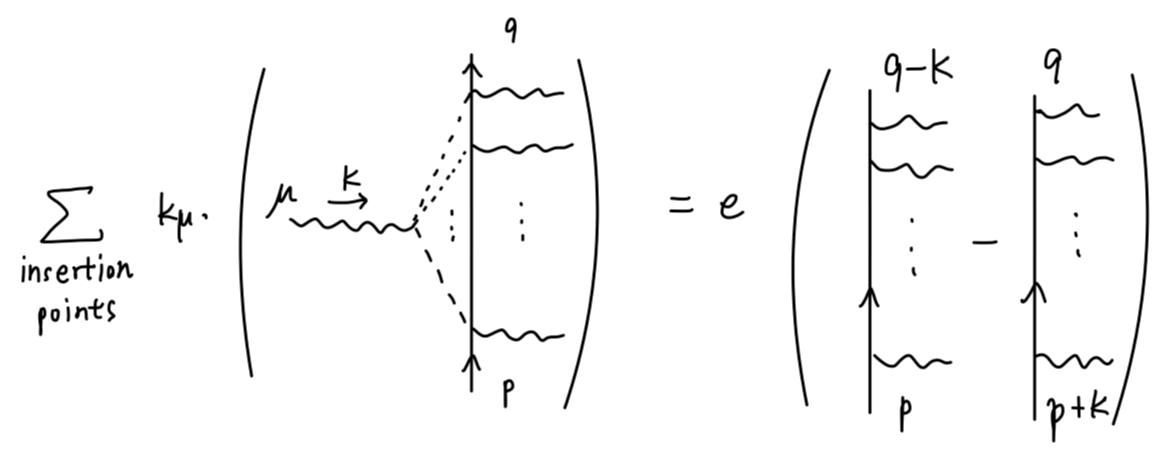$(\frac{i}{\cancel{p}-m})(\frac{i}{\cancel{q}-m})$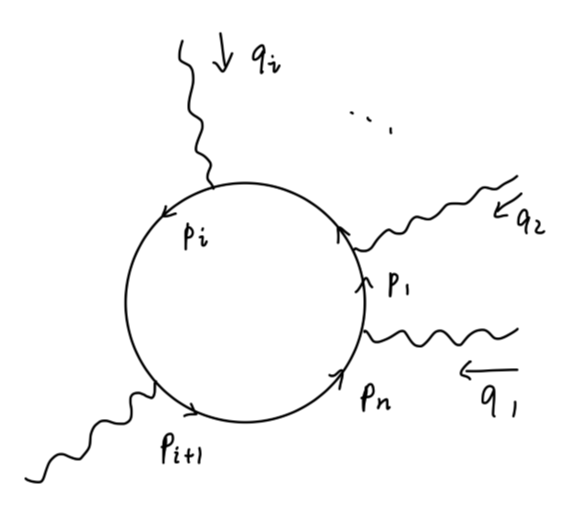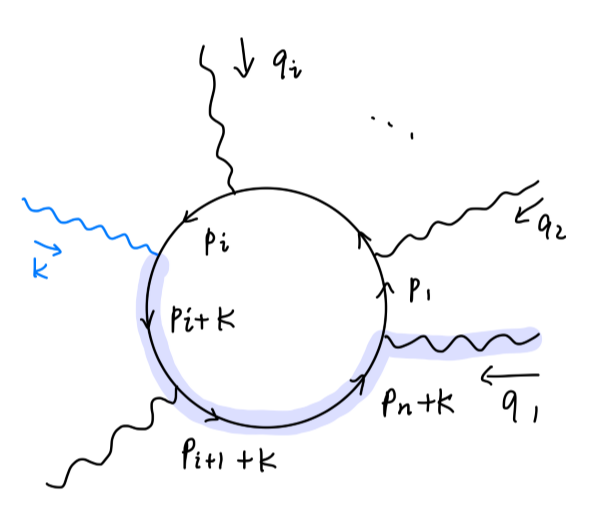$-e\int\frac{d^4p_1}{(2\pi)^4}\mathrm{tr}[(\frac{i}{\cancel{p}_n+\cancel{k}-m})\gamma^{\lambda_n}\cdots(\frac{i}{\cancel{p}_i-m}-\frac{i}{\cancel{p}_i+\cancel{k}-m})\gamma^{\lambda_i}\cdots (\frac{i}{\cancel{p}_1-m})\gamma^{\lambda_1}]$

$-e\int\frac{d^4p_1}{(2\pi)^4}\mathrm{tr}[(\frac{i}{\cancel{p}_n-m})\gamma^{\lambda_n}\cdots(\frac{i}{\cancel{p}_1-m})\gamma^{\lambda_1} - (\frac{i}{\cancel{p}_n + \cancel{k}-m})\gamma^{\lambda_n}\cdots(\frac{i}{\cancel{p}_1 + \cancel{k}-m})\gamma^{\lambda_1}]$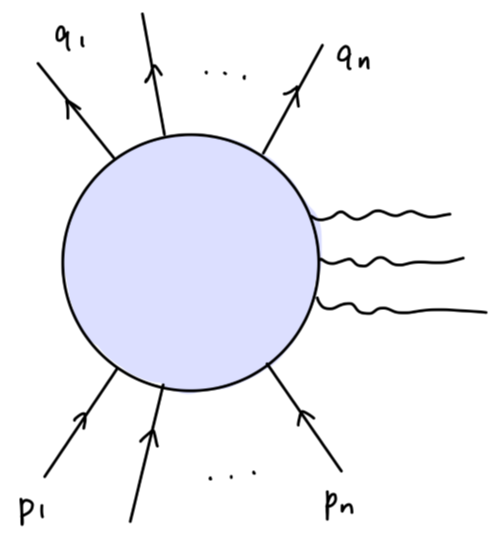$\mathcal{M}_0(p_1\cdots p_n;q_1\cdots q_n)$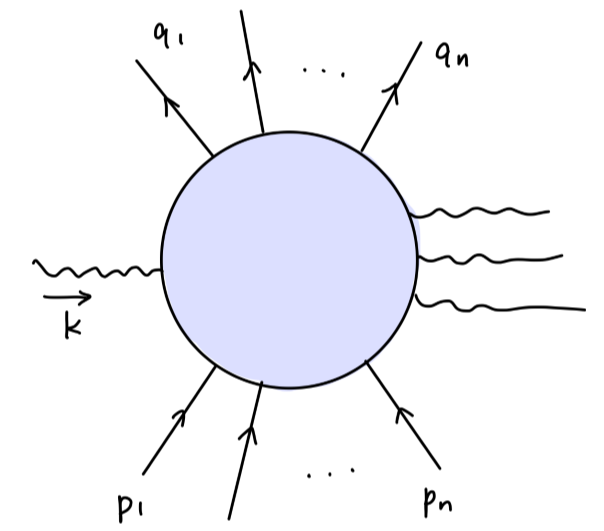$\mathcal{M}(k;p_1\cdots p_n;q_1\cdots q_n)$

$k_{\mu}\mathcal{M}^{\mu}(k;p_1\cdots p_n;q_1\cdots q_n) = e\sum_{i}[\mathcal{M}_0(p_1\cdots p_n;q_1\cdots (q_i-k)\cdots)-\mathcal{M}_0(p_1\cdots (p_i+k)\cdots;q_1\cdots q_n)]$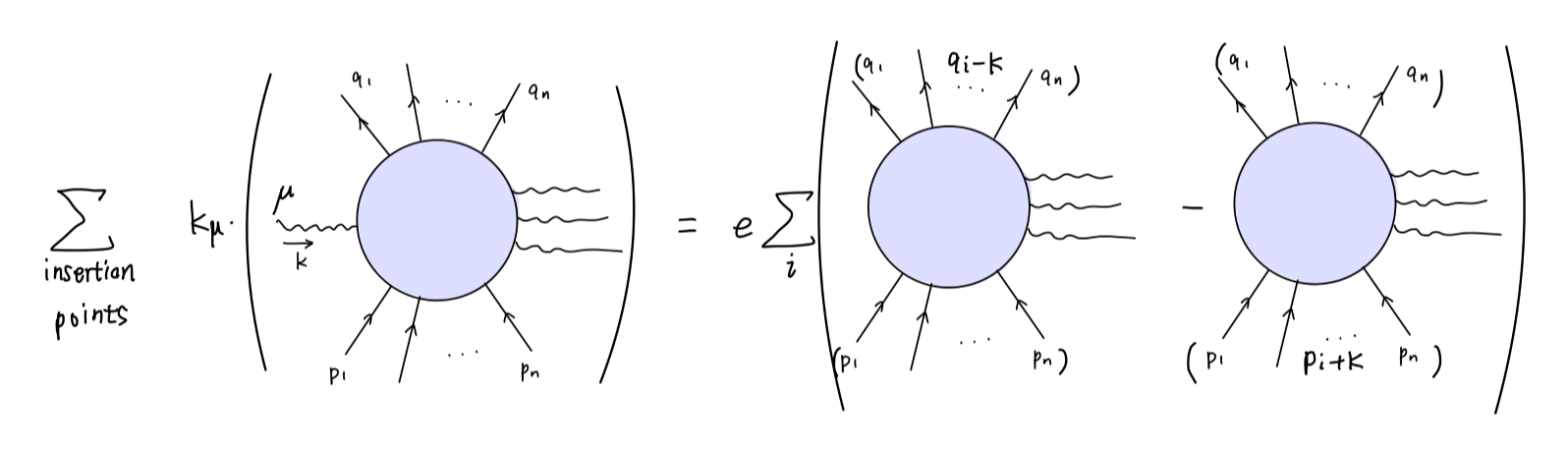$k_{\mu}\mathcal{M}^{\mu} = 0$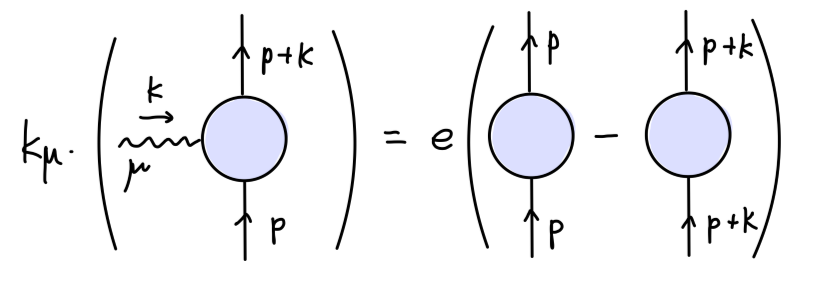$S(p) = \frac{i}{\cancel{p}-m-\Sigma(p)}$

Ward-Takahashi 等式写为：

$S(p+k)(-iek_{\mu}\Gamma^{\mu}(p+k,p))S(p) = e(S(p)-S(p+k))\tag{2}$

$-ik_{\mu}\Gamma^{\mu}(p+k,p) = S^{-1}(p+k)-S^{-1}(p)\tag{3}$

$\Gamma^{\mu}(p+k,p) \rightarrow Z_1^{-1}\gamma^{\mu},\quad k\rightarrow 0\tag{4}$

$S(p) \sim \frac{iZ_2}{\cancel{p}-m}$

$S^{-1}(p+k)-S^{-1}(p) \sim -i\cancel{k}Z_2^{-1},\quad k\rightarrow 0\tag{5}$

$Z_1 = Z_2 \tag{6}$

Ward 等式，流守恒，规范对称性具有相同的含义。Ward 等式是流守恒的图像化描述，规范对称性反映了拉格朗日量的对称性，流守恒则从运动方程角度出发。